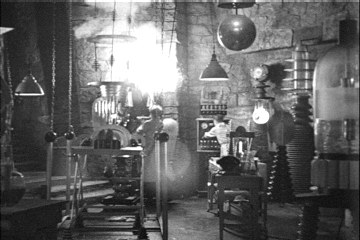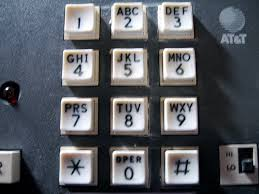# Programmation appliquée en ScalaCopyright © Cay S. Horstmann 2015# Function definition with def

• So far, we always used val to define functions
val triple = (x: Double) => 3 * x
• It is more common to use def:
def triple(x: Double) = 3 * x
• Caution: If def isn't followed by =, you are defining a function returning ()
def triple(x: Double) { 3 * x } // Returns ()!

• With recursive functions, must specify return type
def fac(n: Int):Int = ...

# Recall: Higher-Order Functions

• Functions that take other functions as parameters or that return functions as results are called higher order functions.
• Take the sum of the integers between a and b:
def sumInts(a: Int, b: Int): Int = a.to(b).reduce(_+_)
• Take the sum of the squares of all the integers between a and b :
def square(x: Int): Int = x * x
def sumSquares(a: Int, b: Int): Int = a.to(b).map(square).reduce(_+_)
• Take the sum of the cubes of all the integers between a and b :
def cube(x: Int): Int = x * x * x
def sumCubes(a: Int, b: Int): Int = a.to(b).map(cube).reduce(_+_)
• We can factor out the common pattern of these three functions as following:
def sum(f: Int => Int, a: Int, b: Int): Int = a.to(b).map(f).reduce(_+_)

# Factoring out the parameters

• We use a function that takes another function as parameter.
def sumInts(a: Int, b: Int) = sum(id, a, b)
def sumSquares(a: Int, b: Int) = sum(squares, a, b)
def sumCubes(a: Int, b: Int) = sum(cube, a, b)
• Where
def id(x: Int): Int = x
def square(x: Int): Int = x * x
def cube(x: Int): Int = x * x * x
• We can even be shorter by getting rid of these parameters:
def sum(f: Int => Int): (Int, Int) => Int = (a: Int, b: Int) =>a.to(b).map(f).reduce(_+_)
• sum is now a function that returns another function. The returned function applies the given function parameter f and sums the results.

# What Is ...

sum(square)(1,4)
1. A syntax error—the argument to sum must be a paire of Ints
2. Is equivalent to sumSquares(1,4)
3. Is equivalent to sum(1,4)(square)
4. Returns 30

# Currying

• We can then define:
def sumInts = sum(id)
def sumSquares = sum(square)def sumCubes = sum(cube)
• These functions can in turn be applied like any other function:
sumCubes(1, 10) + sumSquares(10, 20)
• With the last definition of sum we can also call the functions as following
 sum (cube) (1, 10)
• sum(cube) applies sum to cube and returns the sum of cubes function.
• sum(cube) is therefore equivalent to sumCubes.
• This function is next applied to the arguments (1, 10).
• Generally, function application associates to the left:
 sum(cube)(1, 10)   ==   (sum (cube)) (1, 10)
• This style of definition and function application is called currying, named for its instigator, Haskell Brooks Curry (1900-1982), a twentieth century logician.

# Application of Currying: Type Inference

• Seq[A].corresponds(t: Seq[B])(p: (A, B) => Boolean) checks whether two sequences have “corresponding” elements, as defined by p
• For example,
val s = "Mary had a little lamb".split(" ")
val t = Array(4, 3, 1, 6, 4)
s.corresponds(t)((a: String, b: Int) => a.length == b)

• Or more concisely
s.corresponds(t)(_.length == _)
• Why the currying
s.corresponds(t)(_.length == _)
• Otherwise, type inference wouldn't work. The type of t is needed to infer the type B

# Folding

• The product of the elements in Vector(a, b, c) is

a * b * c = 1 * a * b * c = ((1 * a) * b) * c

• Use foldLeft:
def prod(lst: Vector[Int]) = lst.foldLeft(1) (_ * _)
      *
/ \
*   c
/ \
*   b
/ \
1   a
• The first argument of the folding function is the partial answer; the second is the new list value to be considered
• The foldRight operator works right-to-left: a * (b * (c * 1))
• That's useful for right-associative operators (e.g. list construction)

# What Is ...

1.to(10).foldLeft("")(_ + _)
1. "12345678910"
2. "10987654321"
3. 55
4. Something else (syntax error, other result, ...)

# More about Folding

• We could have computed the multiplication function with reduce
• But reduce only works with operators (A, A) => A
• foldLeft works with any operator (B, A) => B
• Remember this from the last lab?
augment(augment(augment(Vector(Vector("1")), '2'), '3'), '4')
• We couldn't use reduce because augment takes arguments of different types
val augment = (a: Vector[Vector[String]], t: Char) => ...
• It's easy with foldLeft—see lab

# Folding and Recursive Functions

• Any recursive definition of the form

f(n) = {(s , if n = 0),(t(f(n - 1), n) , if n > 0):}

can be computed with a fold.
1.to(n).foldLeft(s)(t)
• Example: Factorial (s = 1, t = \_ * \_)

n! = {(1 , if n = 0),((n - 1)! * n , if n > 0):}

• Example: Subsets of { 1 ... n }

S(n) = {({} , if n = 0),(S(n - 1) ∪ { s ∪ {n} | s ∈ S(n - 1) } , if n > 0):}

# Folding and Accumulation

• Task: Get letter frequencies of a string
• E.g. "Mississippi"Map(M -> 1, i -> 4, s -> 4, p -> 2)
• Can update a mutable map of counters—but not with our Scala subset ☺
• How about an immutable map? Produce a new map in each step
                .
.
.
op
/ \
op 's'
/ \
op 'i'
/ \
empty map 'M'

• What is op?
(m, c) => m + (c -> (m.getOrElse(c, 0) + 1))

# Lab• You work with a buddy
• One of you (the coder) writes the code, the other (the scribe) types up answers
• When you get stuck, ask your buddy first!
• Switch coder/scribe roles each lab
• The coder submits the worksheet. Include the scribe's name in the worksheet!
• The scribe submits answers. Include the coder's name in the report!

# Part 1: Currying

1. Here is a function of computing the “maximum” string in a list that works for any ordering.
def max(lst : Seq[String], less : (String, String) => Boolean) =
lst.reduce((x, y) => if (less(x, y)) y else x)

Make a call to max that yields the longest string in a sequence of strings. Important: Use _ for the string parameters in your less function.

2. Now we'll make this generic. Don't worry—it won't hurt a bit:
def max[T](lst : Seq[T], less : (T, T) => Boolean) =
lst.reduce((x, y) => if (less(x, y)) y else x)

What happens when you call max(lst, _ < _)?

3. Ok, that didn't work so well. Currying to the rescue. Curry the max[T] function, exactly like mul2 above. What is the code for your revised function? What happens when you call max2(lst)(_ < _)?
4. Why does the second approach work with _ parameters?

# Part 2. Know When To Fold

1. How do you compute n! with a fold?
val fac = (n: Int) => ...
2. How do you compute n! with a reduce?
3. How do you compute n! with a recursive function?
4. Now on to those subsets where your eyes probably glazed over. We want subsets(2) to be Vector(Vector(), Vector(1), Vector(2), Vector(1, 2)). How do you get that out of subsets(1), i.e. Vector(Vector(), Vector(1))?
5. Ok, what's that in Scala? You are given a vector of vectors s and an integer n, and you want s together with the sequence obtained by adding n to all elements of s. Write a function that does that, and test it.
6. Now write subsets by using foldLeft.
7. It is also easy to write subsets as a recursive function. Try it!
8. Complete and test the letterFrequencies function from the slide 12. What do you get for letterFrequencies("Mississippi")?

# Part 3. Finish Phone Mnemonics1. Last time, we got stuck with repeatedly calling augment to get all ways of breaking up a string into substrings. For example, here are all substrings of "1234"
augment(augment(augment(Vector(Vector("1")), '2'), '3'), '4')
Get last week's worksheet from your buddy and copy over all you need to today's worksheet.
2. Now we want this to work for arbitrary strings, not just "1234". Of course, we want to use foldLeft with augment. What is the seed value? And to what sequence do you apply foldLeft?
3. Implement val substrings = (s: String) => .... What is substrings("2728")?
4. Note that's a vector of Vector[String]. The shining achievement of last week's lab was the function wordsForDigitSequence that found all words for such a sequence. What do you get when you call
substrings("2728").map(wordsForDigitsSequence)
5. Ok, that's not quite what we want. How do you fix it?
6. Now implement val phoneMnemonics = (digits: String) => ... that does this for any string of digits, not just "2728". What is phoneMnemonics("7225247386")? (You can change the cutoff limit of the worksheet in the preferences to see all solutions.)